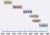# Introduction to Classical Mechanics

Free!

language English Optional, paid
Category:

This introductory course on Classical Mechanics covers the following topics: Euler Lagrange Equations, Small Oscillations, Central Force Problem, Rigid Body Motion.

COURSE LAYOUT

Week 1:Generalised coordinates, D’ Alembert’s Principle, Euler Lagrange equation of motion and its applications.
Week 2:Hamilton’s Principle. Conservation laws.
Week 3: Small oscillations: Free Oscillations, Damped oscillations.
Week 4:Forced Oscillations, Resonance, Normal Coordinates.
Week 5:Central force problem, reduction to 1 body problem, Equation of motion and first integrals.
Week 6:Classification of orbits. Scattering in central field.
Week 7: Kinematics of rigid body motion: Degrees of freedom of rigid body, orthogonal transformations.
Week 8:Euler angles, Euler’s theorem.
Week 9:Finite and infinitesimal rotations.What are tensors? Moment of inertial tensor
Week 10:Principle axis transformation,Euler Equation of motion
Week 11: Torque free motion of a rigid body. Heavy symmetrical top with one point fixed.
Week 12:Hamilton equation of motion, Conservation theorems, Canonical transformations.

## User Reviews

0.0 out of 5
0
0
0
0
0

There are no reviews yet.

×